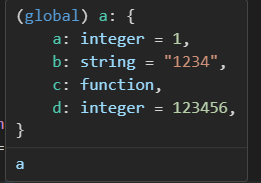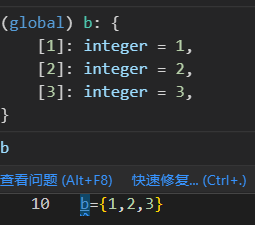## 【Lua学习笔记】Lua入门

article2023/10/2 20:33:21/### 文章目录

• Lua变量
• 数据类型
• 变量声明
• 其他表示
• Lua语法
• 判断
• 逻辑判断（Lua很特殊，这个比较重要）
• 短路判断
• if
• if else
• 循环
• while
• for
• repeat
• 迭代器
• 泛型for迭代器
• 无状态迭代器
• 多状态的迭代器
• Lua函数
• select方法
• 数组
• 字符索引
• _G

（不是教程，推荐有编程基础的人观看本文）

``````function start()
``````

# Lua变量

## 数据类型

nil只有值nil属于该类，表示一个无效值（在条件表达式中相当于false）（类似与Null或者None）
booleanfalse和true
number双精度浮点数
string字符串由一对双引号或单引号来表示
function由 C 或 Lua 编写的函数
userdata表示任意存储在变量中的C数据结构
tableLua 中的表（table）其实是一个"关联数组"（associative arrays），数组的索引可以是数字、字符串或表类型。在 Lua 里，table 的创建是通过"构造表达式"来完成，最简单构造表达式是{}，用来创建一个空表。

## 变量声明

``````a = 5               -- 全局变量
local b = 5         -- 局部变量
``````

``````a               -- nil
a = 5               -- number
a = false         -- bool
a = ""         -- string,单双引号都可以,支持转义字符,print出空字符串而非nil

s = string.char(0x30,0x31)
n = string.byte(s,2)  --转为Ascii码，第一个字符是从1开始的而非0
print(s,n)     --结果: 01  49
a,b,c = 1,"a"         -- 多重赋值
print(a, b, c)         -- 1  a  nil
a = nil			 -- nil,回收变量
``````
``````local multilineString = [[
This is a multiline string.
It can contain multiple lines of text.
No need for escape characters.
]]
print(multilineString)    -- 按格式输出括号内字符
This is a multiline string.
It can contain multiple lines of text.
No need for escape characters.
``````

Lua 对多个变量同时赋值，不会进行变量传递，仅做值传递：

``````a, b = 0, 1
a, b = a+1, a+1
print(a,b);               --> 1   1而非1	  2
``````

## 其他表示

``````a = 0x11    -- 16进制表示,16+1=17
b = 2e10    -- 科学计数法,2*(10^10)
c = 5^2		-- 乘方,25
d = 5%2		-- 取余(mod),1
e = 5//2		-- 整除运算,2 (>=lua5.3)
f = 1<<3	-- 移位符号(>=lua5.3),二进制表示下移位:1 = 00000001左移三位(000)00001000 = 8
a,b = "abc","def"
c= a..b  --字符串连接,"abcdef"
print(#c) --#输出变量长度（可以是字符串或者表）,6
-- 其他语法例如tostring(number),tonumber(string)都是很常规的
n = tonumber("a")   --nil,需要注意的是lua和cpp不同,单个字符也不会转为ASCII码
``````

# Lua语法

## 判断

### 逻辑判断（Lua很特殊，这个比较重要）

Lua认为false和nil为假，true和非nil为真,所以0代表也是真。

``````a = nil -- false
b = 0  -- true
print(a and b)
print(a or b)
print(not a)

nil
0
true
``````

（例如a=nil，值对应false，所以a and b 返回了nil。而b=0 值对应True,a or b返回了0）

``````a = nil -- false
d = false -- false

B = 0   -- true
C = 1   -- true
and时一真一假输出结果是false的一方
print(a and C) --逻辑判断值返回了逻辑符号左右值中的对应真值和假值
print(C and d)
or时一真一假输出结果是true的一方
print(a or C)
print(C or d)

nil
false

1
1

``````
``````以下例子请抛弃依次执行语句的程序思维,使用更逻辑化,更符合计算机的思维来判断
a = nil -- false
d = false -- false

B = 0   -- true
C = 1   -- true
and时两侧都是真值，则返回右侧的值
print(B and C)
print(C and B)
and时两侧都是假值，则返回左侧的值
print(a and d)
print(d and a)

1
0

nil
false

or时两侧都是真值，则返回左侧的值
print(B or C)
print(C or B)
or时两侧都是真值，则返回右侧的值
print(d or a)
print(a or d)

0
1

nil
false
``````

``````接下来用T代表真，F代表假，?代表任意bool
print(F and ?)

print(T and ?)

print(T or ?)

print(F or ?)
or运算中如果左值为false,最后结果则是由?是true还是false决定,因此输出结果一定是右值

``````

#### 短路判断

``````基于上述的原理我们可以进行短路判断
a = nil -- false
b = 0  -- true
print(b > 10 and "yes" or "no")

no

【T and A or B = A, F and A or B =B】

``````

### if

``````if(0)
then
print("0 为 true")
end
``````

### if else

``````a = 100;
if( a < 20 )
then
print("a 小于 20" )
else
print("a 大于 20" )
end
print("a 的值为 :", a)  --a 大于 20
--a 的值为 :    100
``````

## 循环

### while

break 关键字是通用是

``````while( a < 20 )
do
a = a+1
end
``````

### for

``````for i=1,10,2 do  --- 三个值依次代表起始,结束,步长,步长不输入默认为1
print(i)
end
``````

### repeat

``````a = 10
repeat  -- 不同与while for,repeat在循环结束时判断,类似do while
print("a的值为:", a) --输出10,11,12,13,14,15
a = a + 1
until( a > 15 ) --当a=16时退出
``````

## 迭代器

### 泛型for迭代器

``````array = {"Google", "Runoob"}

for key,value in ipairs(array)  -- key,value自定义常量名,ipairs(array)迭代列表
do
print(key, value)
end

2  Runoob
``````

### 无状态迭代器

``````function square(iteratorMaxCount,currentNumber)
if currentNumber<iteratorMaxCount
then
currentNumber = currentNumber+1
return currentNumber, currentNumber*currentNumber
end
end
--定义了一个方法square实现循环三次后结束

for i,n in square,3,0  --square,3,0代表了迭代方法和它的两个入参,当方法结束主动闭包
do
print(i,n)
end

1    1
2    4
3    9
``````

### 多状态的迭代器

``````array = {"Google", "Runoob"}

function elementIterator (collection)
local index = 0
local count = #collection
-- 闭包函数
return function ()
index = index + 1
if index <= count
then
--  返回迭代器的当前元素
return collection[index]
end
end
end

for element in elementIterator(array)
do
print(element)
end

Runoob
``````

# Lua函数

``````----------------------
function f()  --同样未用local声明,该函数为全局函数
a,b=1,2   --  函数内定义没有local声明也是全局变量
print(a,b)
end

f()		-- 1  2
--------------------
local function f(a,b,n)  --local声明后为局部函数
c, d = a, b
print(c, d,n)
end
f(1,2)		-- 1  2  nil
-------多返回值--------------------
function f(a,b,c)
return a,b,c
end
local i,j =f(1,2)
print(i,j)		-- 1  2
--------可变参数---------------
function average(...)  --使用...可以接收任意多参数
result = 0
local arg={...}    --> arg 为一个table，局部变量
for i,v in ipairs(arg) do
result = result + v
end
print("总共传入 " .. #arg .. " 个数")
print("总共传入 " .. select("#",...) .. " 个数")   --select("#",...)来获取可变参数的数量,和直接#arg功能是一样的
return result/#arg
end

print("平均值为",average(10,5,3,4,5,6))
---总共传入 6 个数
---总共传入 6 个数
---平均值为    5.5
-----------
function average(a,...)  --也可以用固定参数+可变参数,但是固定参数一定放在可变参数之前
end
------------------------
``````

## select方法

select(‘#’, …) 返回可变参数的长度。
select(n, …) 用于返回从起点 n 开始到结束位置的所有参数列表。

``````function cal_sum(...)
a,b =select(3,...)
print(select(3, ...))
print(select("#",...) )
end
cal_sum(1,2,3,nil,5,5)
print(a,b)

3	nil	5	5
6
3	nil

``````

# 数组

Lua中没有传统意义的单一类型的数据结构，只有一种table结构，它能顺序存放里面的所有值，并提供了索引以供访问。

``````function P ()
return 1;
end
a={1,"ac",{},function() end,P}
print(a)
print(a)
print(a)
print(#a)
a = 1223;
print(a)
print(#a)

table: 00BCA580
function: 00BCCAC8
function: 00BCCC48
5
1223
6
``````

``````-- insert用法,没有返回值
table.insert(a, "d") --插入到尾部
print(a)
s = table.insert(a, 2, 233) --插入到位置2,其后的元素都会后移一位
print(s)
print(a)

d
nil
233
``````
``````-- remove会有返回值
s = table.remove(a, 1)
print(s)
print(a)

1
ac
``````

## 字符索引

table可以以字符作为索引：

``````a = {
a = 1,
b = "1234",
c = function()
end,
d=123456,
["!?"] =123
}
a["abc"]="abc"
print(a["a"])
print(a.a)
print(a["!?"])   -- 这只是个例子，别用奇怪的符号命名
-- print(a.!?)   --打印不了的,符号会报错
print(a.abc)
print(a.def)    --没有这个下标

1
1
123
abc
nil
``````## _G

_G是一个全局table，它储存了所有的全局变量,我们可以通过它来访问任意全局变量

``````a = 1
b = 2
print(_G["a"])
print(_G.b)

1
2
``````

``````之前我们往tableA里不是使用table.insert插入了一个值吗？让我们看看怎么实现的

table.insert(table, 1, "aa")
- lua内有一个全局变量,它的名字就叫table,它的类型是table
- 我们可以直接使用table.insert的原因其实是直接访问了这个名称为table的table的一个字符串索引
- 这个字符串索引的名称是"insert",这个索引对应的值是一个function
- 这个函数实现了往指定table的指定位置插入指定值的功能
print(table)
print(_G["table"]["insert"])

aa
function: 00CE8B48
``````

``````end
``````

### 13、PHP面向对象2（方法的访问控制、子类继承、常量）

1、类中的方法可以被定义为公有&#xff0c;私有或受保护。如果没有设置这些关键字&#xff0c;则该方法默认为公有。 public定义的方法&#xff0c;可以在类外使用。 protected定义的方法&#xff0c;只能在本类或子类的定义内使用。 private定义的方法&#xff0c;只能在本…

### 【Java中间件】RocketMQ

RocketMQ 一、MQ概述 Message Queue&#xff0c;是一种提供消息队列服务的中间件。提供了消息生产、存储、消费全过程API的软件系统。 MQ的作用 限流削峰&#xff1a;当用户发送超量请求时&#xff0c;将请求暂存&#xff0c;以便后期慢慢处理。如果不使用MQ暂存直接请求到…

### 路由的配置

1、在router中设置路由导航跳转函数,在index.js文件中写这句话&#xff1a; 1.1 只要发生跳转, 就会调用这个函数&#xff1a; 1.2 导航的声明函数 2、访问系统访问控制系统如何形成 3、来一个导航守卫的案例&#xff1a;看看导航守卫的案例&#xff0c;写一个Main.Vue 和login…

### Lab———Git使用指北

Lab———Git使用指北 &#x1f916;:使用IDEA Git插件实际工作流程 &#x1f4a1; 本文从实际使用的角度出发&#xff0c;以IDEA Git插件为基本讲述了如果使用IDEA的Git插件来解决实际开发中的协作开发问题。本文从 远程仓库中拉取项目&#xff0c;在本地分支进行开发&#x…